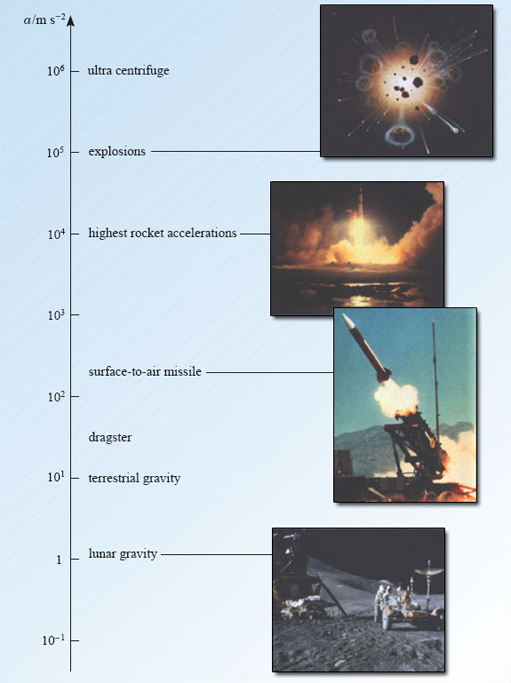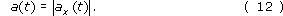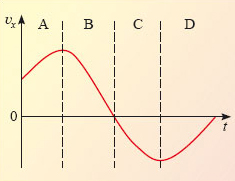Describing motion along a line

This free course is available to start right now. Review the full course description and key learning outcomes and create an account and enrol if you want a free statement of participation.

Free course

4.2 Instantaneous acceleration

The procedure of Question 15 for determining the instantaneous velocity of the car can be carried out for a whole set of different times and the resulting values of v﻿﻿x can be plotted against t to form a graph. This has been done in Figure 28, which shows how the velocity varies with time. At time t = 0 s, the car has zero velocity because it starts from rest. At later times, the velocity is positive because the car moves in the direction of increasing x. The velocity increases rapidly at first, as the car picks up speed. Subsequently, the velocity increases more slowly, and eventually the car settles down to a steady velocity of just over 3 m s−1. We have already come across graphs of this general type in Section 3; they are known as velocity-time graphs. The crucial new feature here is that the velocity now depends on time.Figure 28: A velocity-time graph for the moving car

The time dependence of velocity can have dramatic consequences. If you are on board a train, moving at a constant velocity, you might not even be aware of your motion and you will have no difficulty in, say, drinking a cup of coffee. However, drinking coffee can become distinctly hazardous if the driver suddenly changes the velocity of the train by putting on the brakes. In such situations the important physical quantity is the rate of change of velocity with respect to time, as measured by the gradient of the velocity-time graph. This is the quantity that we usually call acceleration, though once again it should more properly be called instantaneous acceleration. Thus

acceleration at time t = rate of change of velocity with respect to time at time t.

or, if you prefer

acceleration at time t = gradient of velocity-time graph at time t.

Acceleration is a key idea in physics. It was Newton's recognition of the crucial role that acceleration played in determining the link between motion and force that formed the centrepiece of the Newtonian revolution. The detailed study of that revolution would take us too far from our present theme. For the moment let's concentrate on some basic questions about acceleration itself. In particular, in what units should acceleration be measured, and what are typical values of acceleration in various physical contexts? The first of these you can answer for yourself.

Question 15

What are suitable SI units for the measurement of acceleration?

Since acceleration is the rate of change of velocity with respect to time, the units of acceleration are the units of velocity (m s﻿−﻿1﻿) divided by the units of time (﻿s﻿), so acceleration is measured in metres per second per second, which is abbreviated to m s﻿−﻿2.

As for typical values of acceleration, some physically interesting values are shown in Figure 29.Figure 29: Some physically interesting values of acceleration

When dealing with non-uniform motion along the x-axis, the symbol a﻿x﻿(﻿t﻿) is normally used to denote instantaneous acceleration. As usual, a﻿x﻿(﻿t﻿) will be positive if the velocity is increasing with time, though, as you will see below, this statement needs careful interpretation. In contrast to the relationship between velocity and speed, there is no special name for the magnitude of an acceleration, though we shall use the symbol a﻿(﻿t﻿) for this quantity, so we may writeIn physics, the concept of acceleration is precise and quantitative. It is important to realise that this precise definition differs, in some respects, from everyday usage. In ordinary speech, 'accelerating' is a synonym for 'speeding up'. This is not true in physics. In physics, a particle accelerates if it changes its velocity in any way. A particle travelling along a straight line may accelerate by speeding up or by slowing down. It is tempting to suppose that a positive acceleration corresponds to speeding up and a negative acceleration corresponds to slowing down, but this is not always true either, as the following exercise shows.Figure 30: The velocity-time graph used in Question 16

Question 16

The velocity-time graph of Figure 30 is divided into four regions, marked A-D.

(a) In which regions does the particle move in the direction of increasing x? In which regions is it moving in the direction of decreasing x?

(b) In which regions does the particle speed up? In which regions does it slow down?

(c) In which regions does the particle have positive acceleration? In which regions does the particle have negative acceleration?

(a) The particle moves in the direction of increasing x when its velocity is positive. This occurs in regions A and B. Conversely, the particle moves in the direction of decreasing x when its velocity is negative. This occurs in regions C and D.

(b) The particle is speeding up when the magnitude of its velocity is increasing. This occurs in regions A and C. Conversely, the particle is slowing down in regions B and D.

(c) The particle will have a positive acceleration when the gradient of the velocity-time graph is positive. This occurs in regions A and D. Conversely the acceleration is negative in regions B and C.

So, for a particle with negative velocity, an increase in velocity (a positive acceleration) may result in a decrease in speed. You may think that it is a nuisance for physics to use words in such a non-standard way. However, the definitions of velocity and acceleration given in this course are essential if we are to develop a study of kinematics that is both simple and comprehensive. It does mean, however, that you must be careful in using words like velocity, speed and acceleration. In everyday speech, the word deceleration is used to mean 'slowing down', but this term is seldom encountered in physics since it is already covered by the scientific definition of acceleration.

S207_2